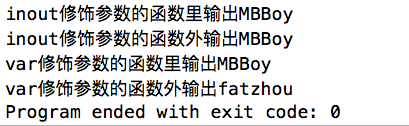# 【原】iOS学习之Swift之语法1(精简版)

## 1. Swift简介

• 2010年的夏天，苹果公司的开发人员Chris Lattne接到了一个特别的任务，为OS X 和iOS平台开发下一代的编程语言，也就是Swift。

• 苹果公司于2014年WWDC（苹果开发者大会）发布的新开发语言Swift，可与Objective-C共同运行于Mac OS和iOS平台，用于搭建基于苹果平台的应用程序。

• 2015年的WWDC开发者大会上，苹果公司推出了Swift2.0版本，并且Swfit完全开源。

• 自从Swift发布以来，在http://www.tiobe.com中的排行一直处于上升的状态。

## 1> 声明变量和常量

• 在Swift中使用 "let" 修饰一个常量，使用 "var" 修饰一个变量;

• let修饰常量的值是不可以更改的；

• var修饰的变量的值是可以更改的；

• 在声明常量和变量的时候可以使用表情符号、中文等命名常量名和变量名。

• Swift定义变量或者常量的时候，需要标识出变量或者常量的类型，如果不标识，会根据初始值自动推断。
// MARK: - 定义常量、变量

// 定义常量（使用完第一次就不能修改它的值了）

// MARK: 定义常量变量：类型的隐式转换

let myGender = "男🐲"

print(myGender)

// 定义变量

var carName = "BMW"

carName = "Audi"

print(carName)

// MARK: 定义常量变量：给定类型

let name : String = "Rose" // 如果给定类型，后面的赋值的时候需要根据类型赋值

let age : Int = 18 // 如果定义常量最好给定初始值，定义变量可以不给初始值

var hobby : Int? // ? 表示hobby的类型为可选类型，其值可以为空

print(hobby)

// 定义一个字符类型的变量
var char : Character = "A" // 赋值字符类型的时候，仅需要一个字母即可

// 定义一个bool类型的变量
var flag : Bool = false
• 值永远不会被隐式转换为其他类型:如果你需要把一个值转换成其他类型，请显式转换
let name = "Angelababy's age is"
let age = 28
let words = name + String(age)

2> 字符串

• String是有序的字符集合，例如“Hello 蓝鸥”

• Swift中的字符串通过String类型表示，当然也可以看成Character字符类型的集合

• \(变量名)输出相关内容【插入式取值】

// MARK: - 字符串string

// (1) 创建一个空的字符串
var str_empty = ""
var str_empty1 = String()

// (2) 判断一个字符串为空字符串
if str_empty.isEmpty {
print("str_empty is empty")
}

// (3) 字符串拼接
var str_url = "http://"
var str = "www.baidu.com"
let str_new_url = str_url + str
print(str_new_url)
print("百度网址：\(str_new_url)") // \(变量名)输出相关内容【插入式取值】

// (4) 获取字符串长度
var str1 = "Hello World"
print("字符串的长度为：\(str1.characters.count)") // 获取字符串的长度

// (5) 字符串的比较
var str2 = "MBBoy"
var str3 = "GBLW"

if str1 != str2 { // ==
print("两个字符串不相等")
}

## 1> 数组

// MARK: - 数组
// Swift中数组中的元素类型必须相同

// 定义一个数组
var nameArray = ["yuanyuan", "GBLW", "WYW"]
print(nameArray)

var nameArray1 : Array<String> = ["YaQian", "XiaoQiang", "FatZhou"]
print(nameArray1)

// 定义一个空数组
let array = Array<String>()

print(array)

// 访问数组的元素
print(nameArray)

// 向数组中添加元素
var int_array = Array<Int>()
int_array.append(520)
int_array.append(123)
print(int_array)

// 使用运算符添加数组元素
int_array += 
int_array += 
print(int_array)

// 使用运算符添加多个数组元素
int_array += [100, 800, 900]
print(int_array)

// 插入元素到具体的位置
int_array.insert(521, atIndex: 1)
print(int_array)

// 移除数组中某个元素
int_array.removeAtIndex(2)
print(int_array)

// 移除所有的元素
int_array.removeAll()
print(int_array)

## 2> 字典

// MARK: - 字典

// 定义一个字典类型的变量
var dict : Dictionary<String,Int> = ["a":18, "b":69, "c":38]
print(dict)

// 定义一个空字典
var dict1 :Dictionary<String,Int> = [:]
var dict2 = Dictionary<String,Int>()
print("\(dict1), \(dict2)")

// 向字典添加元素
dict["d"] = 50
print(dict)

// 修改字典中的元素（根据键去修改值）
dict["a"] = 90
print(dict["a"])

// 删除字典中的内容（根据键去删除）
dict.removeValueForKey("b")
print(dict)

## 4. 元组

1> 概述

• 元组是Swift里面独有的一种数据格式。

• 是一个可以返回多个值的数据格式。

• 在Swift里面声明的时候使用“（）”声明。

• 元组可以通过下标去访问元素，也可以通过key值去访问元素;

• 整体的数据结构有点类似于C语言里面的结构体。

2> 定义一个元组

// 定义一个元组
// 方法一：直接初始化一个元素
let tuples = ("语言", ["iOS", "Java", "html5", "Android"], 12)
print(tuples)

// 方法二：显示初始化
let tuplesNew : (String, Array, Int) = ("语言", ["iOS", "Java", "html5", "Android"], 12)

3> 取出元组中的值

// 取出元组中的值

// 方式1：直接把元组赋值给另一个常量，根据相关标记进行取值
let tuplesNew1 : (type:String, course:Array, number:Int) = tuplesNew
var info = tuplesNew1.type + tuplesNew1.course + String(tuplesNew1.number)
print(info)

// 方式2：直接根据下标取值
var infoNew = tuplesNew.0 + tuplesNew.1 +  String(tuplesNew.2)
print(infoNew)

## 1> 概述

Swift里面的循环结构包含：for、for-in、while、repeat-while

## 2> 循环

// MARK: - 循环

// 第一种形式：
for var i = 0; i < 10; i++ {
print(i)
}

// 第二种形式：
for number in 1..<10 { // 开区间（半闭区间），不包括10
print(number)
}

// 第三种形式：
for number in 1...10 { // 闭区间（全闭区间），包括10
print(number)
}

// while循环和repeat...while循环
var i = 8
while i > 0 {
print(i)
i--
}
repeat {

print("我很好")

}while 1 < 0

## 3> 遍历数组和字典

// 使用循环遍历数组
var animalArray = ["dog","pig","cat","fish"]

for animal in animalArray {
print(animal)
}

// 使用循环遍历字典
var animalDict = ["dog":"🐶", "pig":"🐷"]

for (key, value) in animalDict {
print("\(key) : \(value)")
}

## 1> if分支结构

// if结构
let flag = true
if flag == true {
print("haha")
} else {
print("hehe")
}

## 2> switch结构

在Swift里面，switch的每个case后面会自动的添加一个break，所以不需要手动的去添加了。Swift里面的枚举是可以范围枚举的，系统会根据给定的数据自动的匹配相近的范围

// switch结构
// 特点一：使用fallthrough实现贯穿每种可能
var value = 0
switch value {
case 0:
print(0)
fallthrough
case 1:
print(1)
fallthrough
default:
print("Other")
}
// 特点二：case 后可以使用一个范围
switch value {
case 0...9:
print("小")
case 9...16:
print("大")
default:
print("豹子")
}
// 特点三：case 后可以使用常量和变量
switch value {
case var i where value >= 0 && value < 6: // where 是满足某种条件，可以在分支中进行判断
i = 99
print(i)
default:
print("other")
}
// 特点四：case 后可以匹配一个元组，关键字是元组可以在分支中进行判断
let point = (10, 10)
switch point {
case (10, 0):
print("case1")
case (10, 8):
print("case2")
case (_, 10): // "_" 代表忽略
print("case3")
case (10, 10):
print("case4")
default:
print("case5")
}

注意

• 不需要添加break关键字，也不会贯穿

• 添加fallthrough，可以实现贯穿

• switch要求每个分支必须包含代码

## 7. 枚举

1> 概述

• C 语言中，枚举类型将枚举名和一个整型值相对应。

• Swift 中的枚举更加灵活，不必给每一个枚举成员提供一个确定的值。

• 如果给枚举成员提供一个值（称为“原始”值），则该值的类型可以是字符串，字符，或是一个整型值或浮点数。

2> 代码

// MARK: - 枚举（enum）

// 枚举原始类型为Int类型的
enum Direction:Int {
case east = 0
case south = 1
case west = 2
case north = 3
}
print(Direction.east.rawValue) // rawValue就是找到相对应枚举的原始值

// 枚举原始类型为String类型的
enum Season:String {
case spring = "春天"
case summer = "夏天"
case autumn = "秋天"
case winnter = "冬天"
}
print(Season.autumn.rawValue)

// 根据原始值获取枚举类型
print(Direction(rawValue: 2))

## 8. 函数

1> 概述

• Swift里面用 func 表示声明一个函数。

• Swift的文件格式里面没有类似于OC的.h文件。所以，如果需要定义一个私有的函数，可以在func前面添加private 修饰。

2> 函数定义格式

func 函数名（参数名：参数类型，参数名：参数类型....）->返回值类型 {
函数实现
return 返回值
}

3> 函数类型

• 无参无返回值

// 1. 无返回值，无参数
func fun1() -> Void {
print("无返回值，无参数")
}
fun1()
• 无参有返回值

// 2. 有返回值，无参数
func fun2() -> String {
return "Swift2.0"
}
print(fun2())
• 有参无返回值

// 3. 无返回值，有参数
func fun3(name str:String) -> Void {
print(str)
}
fun3(name: "Swift2.0")
• 有参有返回值

// 4. 有返回值，有参数
func fun4(num1 a:Int, num2 b:Int) -> Int {
return a + b
}
print(fun4(num1: 3, num2: 7))
• 有参多个返回值（返回一个元组）

// 5. 有多个返回值(返回一个元组)
func fun5(num1 a:Int, num2 b:Int) -> (Int, Int, Int) {
return (a + b, a - b, a * b)
}
print(fun5(num1: 69, num2: 38))

3> 外部参数和内部参数

• Swift中，一个参数有两个名字，内部参数名和外部参数名

• 内部参数名：在函数内部使用的参数名称。

• 外部参数名：在函数调用时候，显示的参数名称

// 外部参数和内部参数
// name为外部参数，str为内部参数
func fun6(name str:String) -> Void {
print(str)
}
fun2(name: "Swift2.0")

4> 忽略外部参数

• 默认情况下，函数的第一个参数，不显示外部参数名，其余参数，显示外部参数名，并且和内部参数名一致

• 如果不想要显示外部参数名，可以使用“_”忽略掉

• 注意：函数的第一个参数不能使用“_ ”忽略掉外部参数名。

// 忽略外部参数, "_"的作用是忽略外部参数
func fun7(one:Int, _ two:Int) {
print("忽略外部参数")
}
fun7(8, 9)

5> inout参数

inout参数:

参数默认是用let修饰,即常量

在参数前面添加var,就可以在函数内部修改参数的值,但不能改变实参变量的值.

如果参数使用inout来修饰,就成了输入输出参数,在函数内部修改参数,可以影响实参变量的值.

// inout修饰的参数
func test(inout name:String) {
name = "MBBoy"
print(name)
}

var myName = "zf"
test(&myName)
print(myName)

// 实参的值不变
func test1(var name:String) {
name = "MBBoy"
print(name)
}

var myName1 = "fatzhou"
test1(myName1)
print(myName1)

运行结果：posted @ 2016-05-20 19:16  墨隐于非  阅读(392)  评论(2编辑  收藏  举报